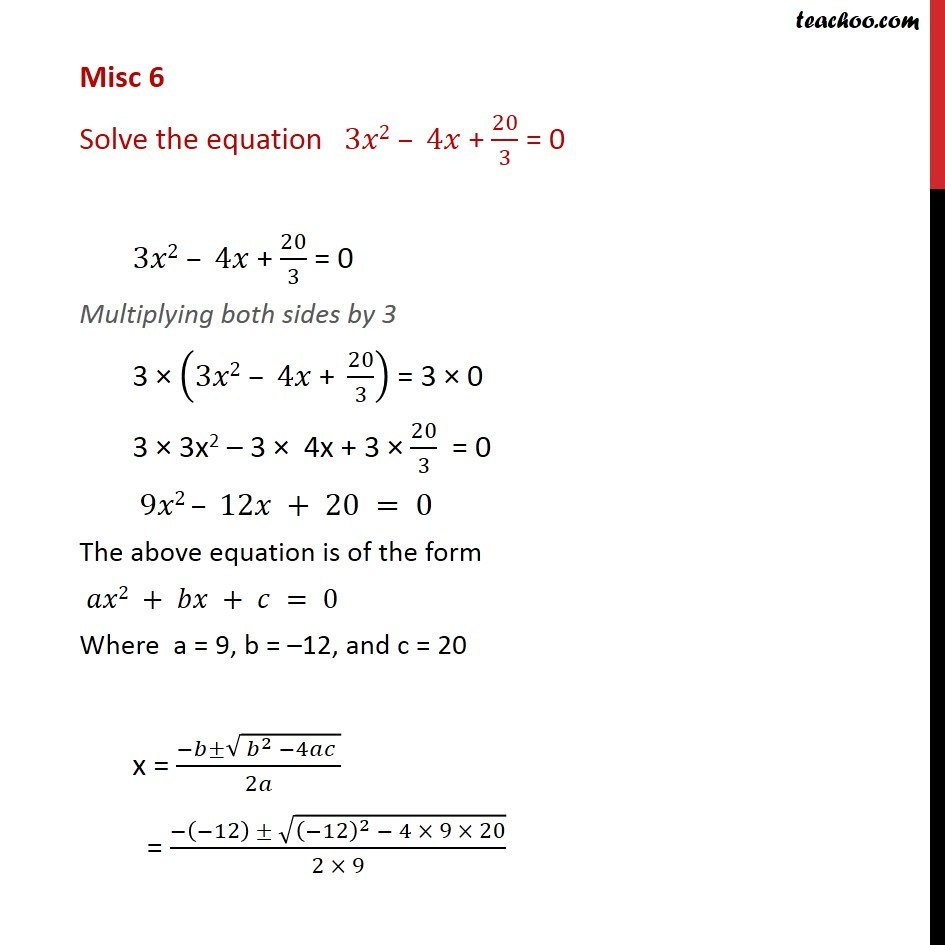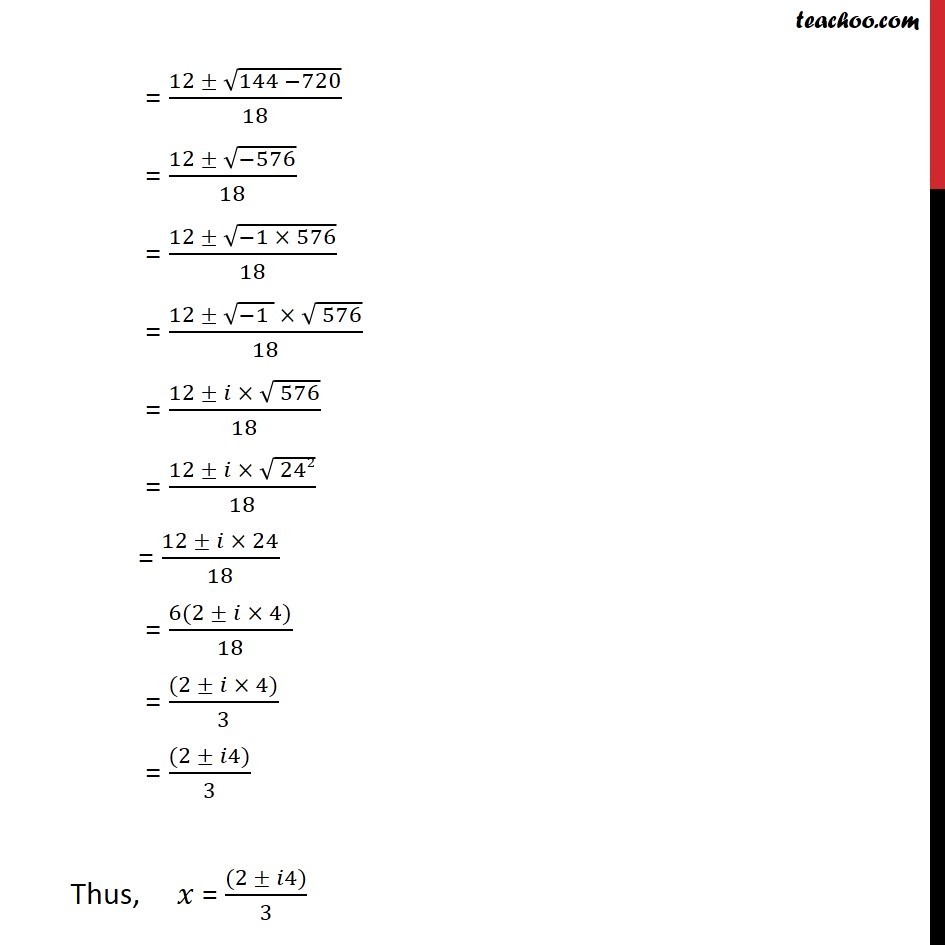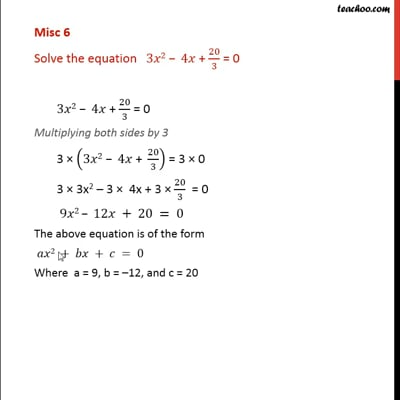Miscellaneous

Chapter 5 Class 11 Complex Numbers (Term 1)
Serial order wiseThis video is only available for Teachoo black users

### Transcript

Misc 6 Solve the equation 3𝑥2 – 4𝑥 + 20/3 = 0 3𝑥2 – 4𝑥 + 20/3 = 0 Multiplying both sides by 3 3 × (3𝑥2 – 4𝑥 "+ " 20/3) = 3 × 0 3 × 3x2 – 3 × 4x + 3 × 20/3 = 0 9𝑥2 – 12𝑥 + 20 = 0 The above equation is of the form 𝑎𝑥2 + 𝑏𝑥 + 𝑐 = 0 Where a = 9, b = –12, and c = 20 x = (−𝑏±√( 𝑏^2 −4𝑎𝑐 ))/2𝑎 = (−(−12) ± √((−12)^2 − 4 × 9 × 20))/(2 × 9) = (12 ± √(144 −720))/18 = (12 ± √(−576))/18 = (12 ± √(−1 × 576))/18 = (12 ± √(−1 ) × √( 576))/18 = (12 ± 𝑖 × √( 576))/18 = (12 ± 𝑖 × √( 242))/18 = (12 ± 𝑖 × 24)/18 = (6(2 ± 𝑖 × 4))/18 = ((2 ± 𝑖 × 4))/3 = ((2 ± 𝑖4))/3 Thus, 𝑥 = ((2 ± 𝑖4))/3